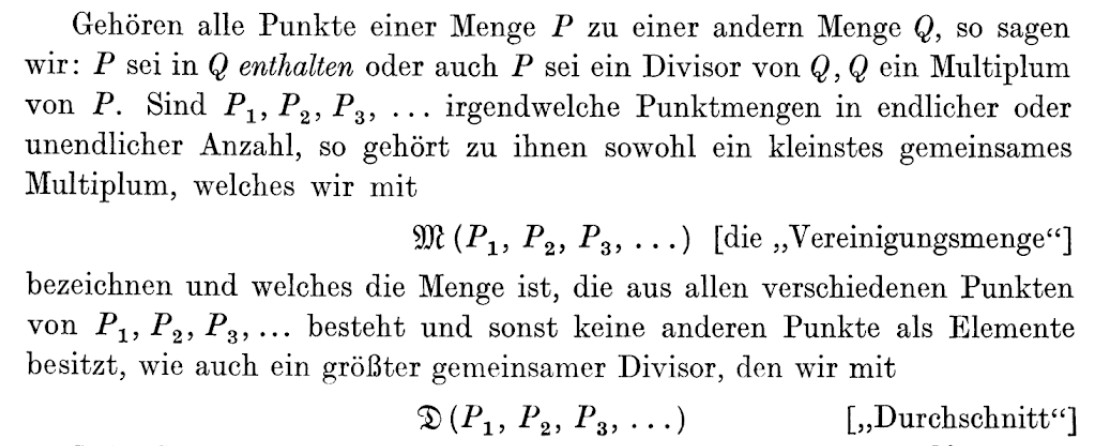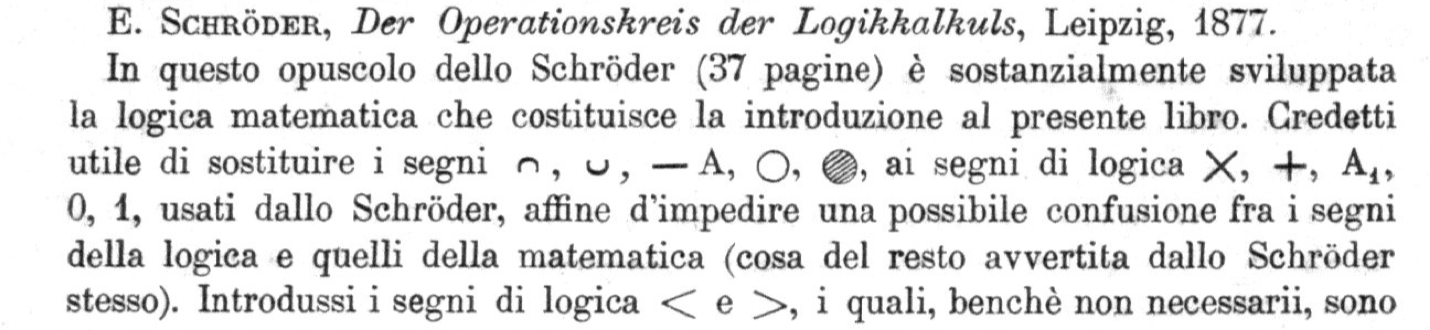# Whence “Durchschnitt” and “Vereinigung”?

Today the set-theoretic operations of intersection $\cap$ [German: Durchschnitt] and union $\cup$ [German: Vereinigung] are standard.

The modern notations are present in the first edition of van der Waerden's Moderne Algebra (1930). However, the notation is mostly missing from Steinitz' Algebraische Theorie der Körper (1910), which was a precursor of van der Waerden. Instead of Menge (for set), Steinitz uses System. The term Durchschnitt is present in Steinitz, but the word Vereinigung and the symbols $\cap,\cup$ are missing.

Has anything been written on the history of set-theoretic language in algebra?

## 1 Answer

An extensive discussion of the origin of "Menge" is given in Earliest Known Uses of Some of the Words of Mathematics (scroll down to "Set and Set Theory"). Cantor's (1880) Über unendliche linear Punktmannigfaltigkeiten is one of the earliest uses. It contains the notation $\{\cdots\}$ for a set and introduces the term "Vereinigung" for the union (symbol ${\cal M}$) and Durchschnitt for the intersection (symbol ${\cal D}$)Schröder (1877) had previously used the notation $+$ and $\times$ for union and intersection. This was changed into the presently used symbols $\cup$ and $\cap$ by Peano (1888) to avoid confusion with addition and multiplication in algebra.In this brief work by Schröder (37 pages) the mathematical logic is developed that forms the introduction of the present book. I found it useful to replace the logical symbols $\times,+,A_1,0,1$ used by Schröder by the symbols $\cap,\cup,-A$, ⚪, ⚫ in order to avoid a possible confusion between symbols from logic and from mathematics (a possible confusion noted by Schröder himself). I also introduced the logical symbols $\lt$ and $\gt$, although not strictly necessary...